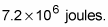##### Physics I For Dummies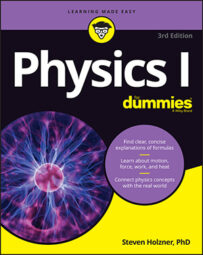In physics, the second law of thermodynamics says that heat flows naturally from an object at a higher temperature to an object at a lower temperature, and heat doesn’t flow in the opposite direction of its own accord.

The law is certainly borne out in everyday observation — when was the last time you noticed an object getting colder than its surroundings unless another object was doing some kind of work? You can force heat to flow away from an object when it would naturally flow into it if you do some work — as with refrigerators or air conditioners — but heat doesn’t go in that direction by itself.

You have many ways to turn heat into work. You may have a steam engine, for example, that has a boiler and a set of pistons, or you may have an atomic reactor that generates superheated steam that can turn a turbine.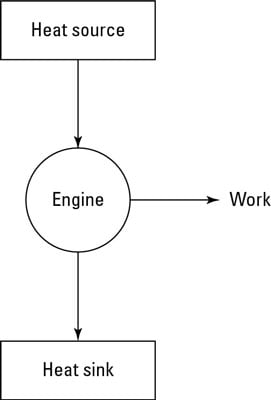A heat engine turns heat into work.

Engines that rely on a heat source to do work are called heat engines; you can see the principle behind a heat engine in the preceding figure. A heat source provides heat to the engine, which does work. The waste heat left over goes to a heat sink, which effectively has an infinite heat capacity, because it can take such a large amount of heat energy without changing temperature. The heat sink could be the surrounding air, or it could be a water-filled radiator, for example. As long as the heat sink is at a lower temperature than the heat source, the heat engine can do work — at least theoretically.

Heat supplied by a heat source is given the symbol Qh (for the hot source), and heat sent to a heat sink is given the symbol Qc (for the cold heat sink). With some calculations, you can find the efficiency of a heat engine. The efficiency is the ratio of the work the engine does, W, to the input amount of heat — the fraction of the input heat that the engine converts to work: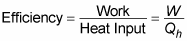If the engine converts all the input heat to work, the efficiency is 1.0. If no input heat is converted to work, the efficiency is 0.0. Often, the efficiency is given as a percentage, so you express these values as 100 percent and 0 percent.

Because total energy is conserved, the heat into the engine must equal the work done plus the heat sent to the heat sink, which means that Qh = W + Qc. Therefore, you can rewrite the efficiency in terms of just Qh and Qc: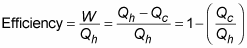Here’s an example: Say that you have a heat engine that’s 78.0 percent efficient and that produces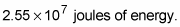Perhaps this is the energy produced by the engine of a car from burning one tank of fuel. (Note: the energy equivalent of 1 gallon of gas is about 1.2 x10^8 J) How much heat does the engine use, and how much does it reject? Well, you know that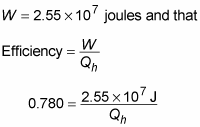Solving for Qh gives you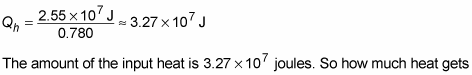left over and sent into the heat sink, Qc? You know that Qh = W + Qc, and you can rearrange the problem to solve for Qc:

Qc = QhW

Plugging in the numbers gives you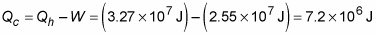The amount of heat sent to the heat sink is